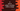# C program to ask the user to select a number within a range### C program to ask the user to select a number within a range :

In this C programming tutorial, we will learn how to ask a user to select a number within a certain range. In this example, we will ask the user to enter a number within 1 and 10. If the number is not in range like 11,0,1,23 etc., we will print one message that it is not valid and the program will ask again to enter a valid number. This program will use one while loop as infinite loop. Means the loop will run continuously till the user will enter one valid number. Let’s take a look at the program :

### C program :

``````#include

int main(){
//1
int selectedNo = -1;

//2
while(1){
//3
printf("Enter a number between 1 and 10 : \n");
scanf("%d",&selectedNo);

if(selectedNo<=1 || selectedNo>=10){
//4
printf("Not a valid no !!\n");
}else{
//5
printf("Entered number is valid.\n");
break;
}
}
}``````

### Explanation of the above program :

The commented numbers in the above program denote the step number below :

1. Create one integer selectedNo to store the current number entered by the user.
2. Start one infinite while loop. while(1) means the code inside this loop will run for infinite amount of time till we forcefully exit from the loop by using break.
3. Inside this loop, ask the user to enter a number between 1 and 10.
4. Now, check if the entered number lies within 1 and 10 or not. If not, print that it is not valid.
5. Else, print that the entered number is valid and exit from the loop using break.

### Sample Output :

``````Enter a number between 1 and 10 :
0
Not a valid no !!
Enter a number between 1 and 10 :
1
Not a valid no !!
Enter a number between 1 and 10 :
12
Not a valid no !!
Enter a number between 1 and 10 :
11
Not a valid no !!
Enter a number between 1 and 10 :
1234
Not a valid no !!
Enter a number between 1 and 10 :
10
Not a valid no !!
Enter a number between 1 and 10 :
3
Entered number is valid.

Enter a number between 1 and 10 :
4
Entered number is valid.``````